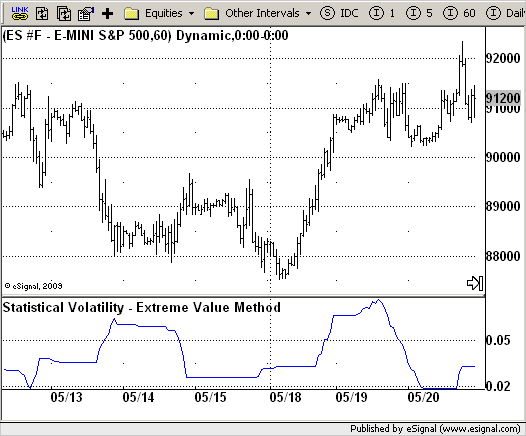# Statistical Volatility - Extreme Value Method

ICE Data Services -

VolatilityExtVal.efs
EFSLibrary - Discussion Board

File Name: VolatilityExtVal.efs

Description:
Statistical Volatility - Extreme Value Method

Formula Parameters:

• nLength : 30

Notes:
This indicator used to calculate the statistical volatility, sometime called historical volatility, based on the Extreme Value Method. Please use this link to get more information about Volatility.

VolatilityExtVal.efsEFS Code:

```/*********************************
Provided By:
eSignal (Copyright c eSignal), a division of Interactive Data
Formula Script (EFS) is for educational purposes only and may be
modified and saved under a new file name.  eSignal is not responsible
for the functionality once modified.  eSignal reserves the right
to modify and overwrite this EFS file with each new release.

Description:
Statistical Volatility - Extreme Value Method

Version:            1.0  05/22/2009

Formula Parameters:                     Default:
nLength                             30

Notes:
This indicator used to calculate the statistical volatility, sometime
called historical volatility, based on the Extreme Value Method.

**********************************/
var fpArray = new Array();
var bInit = false;

function preMain() {
setStudyTitle("Statistical Volatility - Extreme Value Method");
setCursorLabelName("Xtrme Vlty");
var x = 0;
fpArray[x] = new FunctionParameter("nLength", FunctionParameter.NUMBER);
with(fpArray[x++]) {
setLowerLimit(1);
setDefault(30);
}
}

var xVolatilityExtVal = null;

function main(nLength) {
var nBarState = getBarState();
var nVolatilityExtVal = 0;
if (nBarState == BARSTATE_ALLBARS) {
if (nLength == null) nLength = 30;
}
if (bInit ==  false) {
xVolatilityExtVal = efsInternal("Calc_VolatilityExtVal", nLength);
bInit = true;
}
nVolatilityExtVal = xVolatilityExtVal.getValue(0);
if (nVolatilityExtVal == null) return;
return nVolatilityExtVal;
}

var bSecondInit = false;
var xClose = null;
var xMaxC = null;
var xMinC = null;
var xMaxH = null;
var xMinL = null;

function Calc_VolatilityExtVal(nLength) {
var nRes = 0;
var i = 0;
var SqrTime = Math.sqrt(253 / nLength);
if (bSecondInit == false) {
xClose = close();
xMaxC = upperDonchian(nLength, xClose);
xMinC = lowerDonchian(nLength, xClose);
xMaxH = upperDonchian(nLength);
xMinL = lowerDonchian(nLength);
bSecondInit = true;
}
if (xMaxC.getValue(0) == null) return;
nRes = ((0.6 * Math.log(xMaxC.getValue(0) / xMinC.getValue(0)) * SqrTime) +
(0.6 * Math.log(xMaxH.getValue(0) / xMinL.getValue(0)) * SqrTime)) * 0.5;
if (nRes < 0) nRes = 0.0;
if (nRes > 2.99) nRes = 2.99;
return nRes;
}```